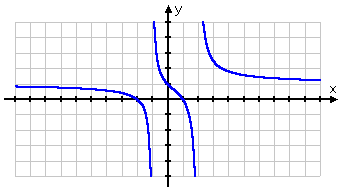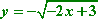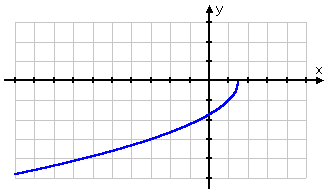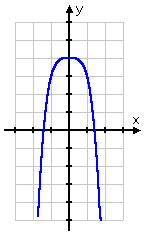# Domain and Range

## Domain and Range of a FunctionDomain:

The domain of a function is the complete set of possible values of the independent variable.

ie;The domain is the set of all possible x-values which will make the function “work”, and will output real y-values.

When finding the domain, remember:
The denominator (bottom) of a fraction cannot be zero.
The number under a square root sign must be positive in this work

eg:
Here is the graph of y=√​x+4​​​:The domain of this function is x≥−4, since x cannot be less than −4. To see why, try out some numbers less than −4 (like −5 or −10) and some more than −4 (like −2 or 8) in your calculator. The only ones that “work” and give us an answer are the ones greater than or equal to −4. This will make the number under the square root positive.

Notes:
The enclosed (colored-in) circle on the point (−4,0). This indicates that the domain “starts” at this point.

How to find the domainIn general, we determine the domainof each function by looking for those values of the independent variable (usually x) which we are allowed to use. (Usually we have to avoid 0 on the bottom of a fraction, or negative values under the square root sign).

Range:The range of a function is the complete set of all possible resulting values of the dependent variable (y,usually), after we have substituted the domain.

ie;The range is the resulting y-values we get after substituting all the possible x-values.
How to find the range:
The range of a function is the spread of possible y-values (minimum y-value to maximum y-value)
Substitute different x-values into the expression for y to see what is happening. (Ask yourself: Is yalways positive? Always negative? Or maybe not equal to certain values?)
Make sure you look for minimum and maximum values of y.
Draw a sketch! In math, it’s very true that a picture is worth a thousand words.

Some Questions:
a) f(x)=x^​2​​+2 Domain: The function f(x) = x^2 + 2 is defined for all real values of x (because there are no restrictions on the value of x).

Hence, the domain of f(x) is”all real values of x”.

Range: Since x^^2 is never negative, x^2 + 2 is never less than 2
Hence, the range of f(x) is”all real numbers f(x)≥2″.

We can see that x can take any value in the graph, but the resulting y = f(x) values are greater than or equal to 2.(b) f(t)=​1/t+2​​
Domain: The function f(t)=1/​t+2​​ is not defined for t = -2, as this value would result in division by zero. (There would be a 0 on the bottom of the fraction.)

Hence the domain of f(t) is”all real numbers except -2″

Range: No matter how large or small t becomes, f(t) will never be equal to zero.

If we try to solve the equation for 0, this is what happens:
0=​t+2​​1​​

Multiply both sides by (t + 2) and we get
0=1

This is impossible.So the range of f(t) is”all real numbers except zero”.

We can see in the graph that the function is not defined for t=−2 and that the function (the y-values) takes all values except 0.C)State the domain and range of the following relation. Is the relation a function?
{(2, –3), (4, 6), (3, –1), (6, 6), (2, 3)}

The above list of points, being a relationship between certain x’s and certain y’s, is a relation. The domain is all the x-values, and the range is all the y-values. To give the domain and the range, I just list the values without duplication:

domain: {2, 3, 4, 6}

range: {–3, –1, 3, 6}

(It is customary to list these values in numerical order, but it is not required. Sets are called “unordered lists”, so you can list the numbers in any order you feel like. Just don’t duplicate: technically, repetitions are okay in sets, but most instructors would count off for this.)

While the given set does represent a relation (because x’s and y’s are being related to each other), they gave me two points with the same x-value: (2, –3) and (2, 3). Since x = 2 gives me two possible destinations, then this relation is not a function.

Note that all I had to do to check whether the relation was a function was to look for duplicate x-values. If you find a duplicate x-value, then the different y-values mean that you do not have a function.

D)State the domain and range of the following relation. Is the relation a function?
{(–3, 5), (–2, 5), (–1, 5), (0, 5), (1, 5), (2, 5)}

I’ll just list the x-values for the domain and the y-values for the range:

domain: {–3, –2, –1, 0, 1, 2}
range: {5}

This is another example of a “boring” function, just like the example on the previous page: every last x-value goes to the exact same y-value. But each x-value is different, so, while boring, this relation is indeed a function. In point of fact, these points lie on the horizontal line y = 5.

There is one other case for finding the domain and range of functions. They will give you a function and ask you to find the domain (and maybe the range, too). I have only ever seen (or can even think of) two things at this stage in your mathematical career that you’ll have to check in order to determine the domain of the function they’ll give you, and those two things are denominators and square roots.

E)Determine the domain and range of the given function:The domain is all the values that x is allowed to take on. The only problem I have with this function is that I need to be careful not to divide by zero. So the only values that x can not take on are those which would cause division by zero. So I’ll set the denominator equal to zero and solve; my domain will be everything else.

x^2 – x – 2 = 0
(x – 2)(x + 1) = 0
x = 2 or x = –1

Then the domain is “all x not equal to –1 or 2”.

The range is a bit trickier, which is why they may not ask for it. In general, though, they’ll want you to graph the function and find the range from the picture.

In this case:As I can see from my picture, the graph “covers” all y-values (that is, the graph will go as low as I like, and will also go as high as I like). Since the graph will eventually cover all possible values of y, then the range is “all real numbers”.

F)Determine the domain and range of the given function:The domain is all values that x can take on. The only problem I have with this function is that I cannot have a negative inside the square root. So I’ll set the insides greater-than-or-equal-to zero, and solve. The result will be my domain:

–2x + 3 > 0
–2x > –3
2x < 3
x < 3/2 = 1.5

Then the domain is “all x < 3/2”.

The range requires a graph. I need to be careful when graphical radicals.The graph starts at y = 0 and goes down from there. While the graph goes down very slowly, I know that, eventually, I can go as low as I like (by picking an x that is sufficiently big). Also, from my experience with graphing, I know that the graph will never start coming back up. Thenthe range is “y < 0”.

G)Determine the domain and range of the given function:
y = –x^4 + 4

This is just a garden-variety polynomial. There are no denominators (so no division-by-zero problems) and no radicals (so no square-root-of-a-negative problems). There are no problems with a polynomial. There are no values that I can’t plug in for x. When I have a polynomial, the answer is always that the domain is “all x”.

The range will vary from polynomial to polynomial, and they probably won’t even ask, but when they do, I look at the picture:The graph goes only as high as y = 4, but it will go as low as I like. Then:

The range is “all y < 4”.

(Visited 541 times, 1 visits today)
error: Content is protected !!